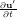### 10.4 Stability analysis Session file configuration

The type of equation which is to be solved is specified through the `EqType` option in the session file. This can be set to any of the following:

 Equation to solve+ L(U,u′) = -∇p + ν∇2u′

 Equation Type Dimensions Projections Algorithms UnsteadyNavierStokes 2D, Quasi-3D Continuous VCS,DS

#### 10.4.1 Solver Info

• `Eqtype`: sets the type of equation to solve, according to the table above.
• `TimeIntegrationMethod`: the following types of time integration methods have been tested with each solver:

 Explicit Diagonally Implicit IMEX Implicit `UnsteadyNavierStokes` X X
• `Projection`: the Galerkin projection used may be either
• `Continuous`: for a C0-continuous Galerkin (CG) projection;
• `Discontinuous`: for a discontinous Galerkin (DG) projection.
• `EvolutionOperator`:
• `Nonlinear` (non-linear Navier-Stokes equations).
• `Direct` (linearised Navier-Stokes equations).
• `Adjoint` (adjoint Navier-Stokes equations).
• `TransientGrowth` ((transient growth evolution operator).
• `Driver`: specifies the type of problem to be solved:
• `Standard` (time integration of the equations)
• `ModifiedArnoldi` (computations of the leading eigenvalues and eigenmodes using modified Arnoldi method)
• `Arpack` (computations of eigenvalues/eigenmodes using Implicitly Restarted Arnoldi Method (ARPACK) ).
• `ArpackProblemType`: types of eigenvalues to be computed (for Driver Arpack only)
• `LargestMag` (eigenvalues with largest magnitude).
• `SmallestMag` (eigenvalues with smallest magnitude).
• `LargestReal` (eigenvalues with largest real part).
• `SmallestReal` (eigenvalues with smallest real part).
• `LargestImag` (eigenvalues with largest imaginary part).
• `SmallestIma` (eigenvalues with smallest imaginary part ).
• `Homogeneous`: specifies the Fourier expansion in a third direction (optional)
• `1D` (Fourier spectral method in z-direction).
• `ModeType`: this specifies the type of the quasi-3D problem to be solved.
• `MultipleMode` (stability analysis with multiple modes).
• `SingleMode` (BiGlobal Stability Analysis: full-complex mode).
• `HalfMode` (BiGlobal Stability Analysis: half-complex mode u.Re v.Re w.Im p.Re).

#### 10.4.2 Parameters

The following parameters can be specified in the `PARAMETERS` section of the session file:

• `Re`: sets the Reynolds number
• `Kinvis`: sets the kinematic viscosity ν.
• `kdim`: sets the dimension of the Krylov subspace κ. Can be used in: `ModifiedArnoldi` and `Arpack`. Default value: 16.
• `evtol`: sets the tolerance of the eigenvalues. Can be used in:
• `imagShift`: provide an imaginary shift to the direct sovler eigenvalue problem by the specified value. lttModifiedArnoldi and `Arpack`. Default value: 10-6.
• `nits`: sets the maximum number of iterations. Can be used in: `ModifiedArnoldi` and `Arpack`. Default value: 500.
• `LZ`: sets the length in the spanswise direction Lz. Can be used in `Homogeneous` set to `1D`. Default value: 1.
• `HomModesZ`: sets the number of planes in the homogeneous directions. Can be used in `Homogeneous` set to `1D` and `ModeType` set to `MultipleModes`.
• `N_slices`: sets the number of temporal slices for Floquet stability analysis.
• `period`: sets the periodicity of the base flow.
• `realShift`: provide a real shift to the direct sovler eigenvalue problem by the specified value.

#### 10.4.3 Functions

When using the direct solver for stability analysis it is necessary to specify a Forcing function “StabilityCoupledLNS” in the form:

1<FORCING>
2   <FORCE TYPE="StabilityCoupledLNS">
3   </FORCE>
4</FORCING>

This is required since we need to tell the solver to use the existing field as a forcing function to the direct matrix inverse as part of the Arnoldi iteration.

Note: Examples of the set up of the direct solver stability analysis (and other incompressible Navier-Stokes solvers) can be found in the regression test directory `NEKTAR/solvers/IncNavierStokesSolver/Tests`. See for example the files `PPF_R15000_ModifiedArnoldi_Shift.tst` and `PPF_R15000_3D.xml` noting that some parameters are specified in the .tst files.